/

### High resolution extended image near field optics 4. Exact radiating solutions to Maxwell’s equations in the presence of idealised plane mirrors

Next page

Consider now the behaviour of inwardly and outwardly radiating (magnetic) dipoles in the presence of an idealised plane mirror, i.e. the solution, say, in the half space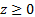arising from a dipole whose origin in Cartesian coordinates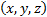is given by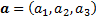(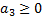) and whose direction is given by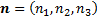if there is:

(a)    A vacuum in the region; and

(b)   A perfectly conducting plane mirror at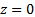.

As Born & Wolf (1980) explain, the exact boundary condition satisfied on the planeis that the component of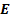tangential tois zero.

Now let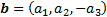and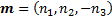. The reason we focus on magnetic rather than electric dipoles using the terminology in Section 3 is that the superposition of two such equal magnitude and in-phase dipoles, one emanating at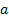pointing in directionand the other emanating at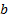and pointing in the direction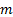then exactly satisfies the required boundary condition at. Suppose we write this superposition as: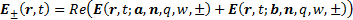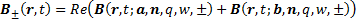It exactly satisfies the boundary condition because atwe have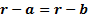and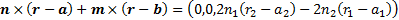, if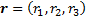in Cartesian coordinates. So the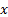and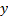components of the electric field atare both zero and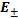is thus exactly perpendicular to the mirror.

Consider further the special case of the above where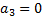and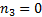. We then have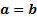and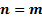, the dipole is emanating from the plane mirror itself and the solutions take the form: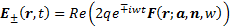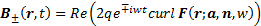Surfaces of constant phase for this special case are hemispheres centred about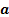. The direction and amplitude of the real physical values ofon each such hemisphere then have the form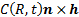, i.e.is perpendicular to both the direction of the corresponding radius vector and the direction of the dipole and has a maximum amplitude proportional to the sine of the angle between these two vectors.

Consider also the situation where we have the special case solution form as above and we place a perfectly conducting metallic hemispherical mirror placed at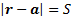(in the region) for some constant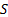. Asis exactly tangential to each such hemisphere, any exact outwardly radiating (magnetic) dipole fromwill strike the hemisphere, be reflected with a 180 degree phase transition and create exactly the right boundary conditions to create an exact inwardly radiating (magnetic dipole).

If the hemisphere was centred at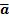, some point on the plane mirror not far from, then outwardly radiating dipoles fromwould not have the right characteristics to generate the exact boundary conditions needed for an equivalent inwardly radiating dipole, at least not one that radiates back to. However, any dipole emanating fromthat bounced a second time off the plane mirror and then of the hemispherical mirror would then have the right characteristics, to first order, to create the required boundary conditions. So, ifis sufficiently large compared to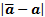then the layout would again create an arbitrarily accurate inwardly radiating (magnetic) dipole with destination.